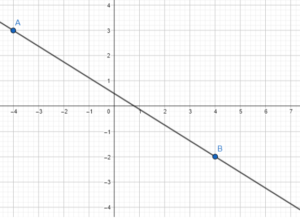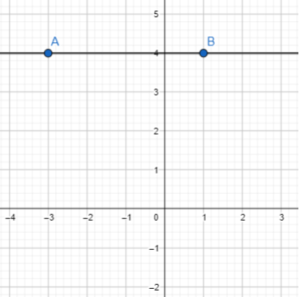# Math in Focus Grade 8 Chapter 4 Lesson 4.2 Answer Key Understanding Slope-Intercept Form

Go through the Math in Focus Grade 8 Workbook Answer Key Chapter 4 Lesson 4.2 Understanding Slope-Intercept Form to finish your assignments.

## Math in Focus Grade 8 Course 3 A Chapter 4 Lesson 4.2 Answer Key Understanding Slope-Intercept Form

Technology Activity

Materials
graphing calculator

EXPLORE THE RELATIONSHIP BETWEEN y = mx AND y = mx + b

Work in pairs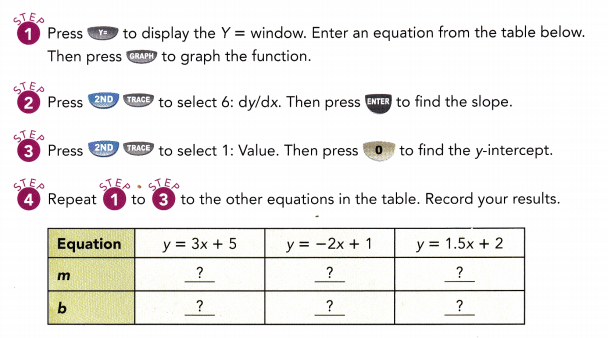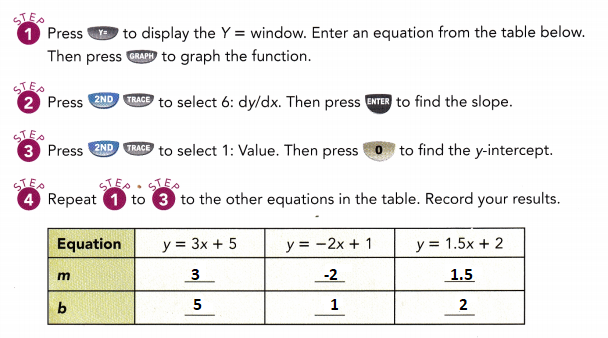Math Journal The equation of another line is given by 2y = 5x – 4. It can also be written as y = 2.5x – 2. Predict the y-intercept. Use the graphing calculator to check your prediction. Is your prediction correct?
Slope m = 2.5
y-intercept = -2

### Math in Focus Grade 8 Chapter 4 Lesson 4.2 Guided Practice Answer Key

Write an equation for each line.

Question 1.
The line passes through the points (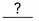,) and (,).
Slope m = $$\frac{?-?}{?-?}$$
= $$\frac{?}{?}$$
=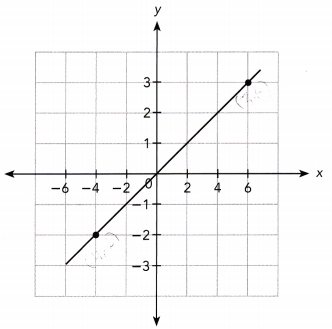The line intersects the y-axis at the point (,).
So, the y-intercept is.
So, the equation of the line is.
The line passes through the points (6, 3) and (-4, -2).
Slope m = $$\frac{6-(-4)}{3-(-2)}$$
= $$\frac{10}{5}$$
= 2
The line passes through the y-axis at the point (0, 0).
Thus m = 2 and y-intercept b is 0.
y = 2x

Question 2.
The line passes through the points (,) and (,).
Slope m = $$\frac{?-?}{?-?}$$
= $$\frac{?}{?}$$
=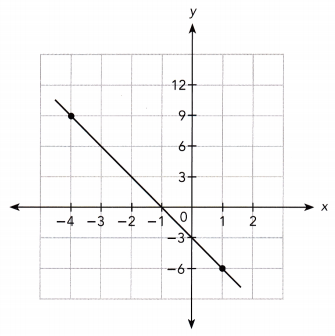The line intersects the y-axis at the point (,).
So, the y-intercept is.
So, the equation of the line is.
The line passes through the points (1, -6) and (-4, 9).
Slope m = $$\frac{9-(-6)}{-4-1}$$
= $$\frac{15}{-5}$$
= -3
The line intersects the y-axis at the point (0, -3).
So, the y-intercept is -3.
So, the equation of the line is y = -3x -3.

Question 3.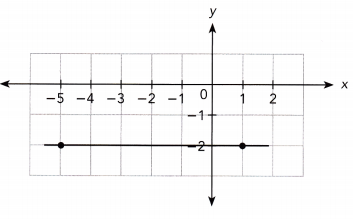The line passes through the points (-5, -2) and (1, -2).
Slope m = $$\frac{-2-(-2)}{1+5}$$
= $$\frac{0}{6}$$
= 0
The line intersects the y-axis at the point (0, -2).
So, the y-intercept is -2.
So, the equation of the line is y = 0x -2.

Question 4.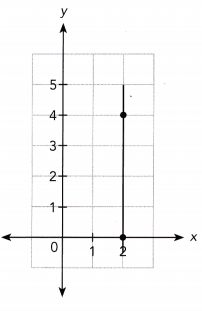The line passes through the points (0, 2) and (2, 4).
Slope m = $$\frac{4-2)}{2-0}$$
= $$\frac{2}{2}$$
= 1
The line intersects the y-axis at the point (0, 0).
So, the y-intercept is 0.
So, the equation of the line is y = -2x + 2

### Math in Focus Course 3A Practice 4.2 Answer Key

Identify the y-Intercept. Then calculate the slope using the points indicated.

Question 1.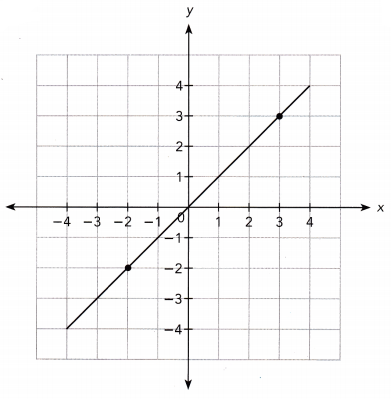The line passes through the points (-2, -2) and (3, 3).
Slope m = $$\frac{3-(-2)}{3-(-2)}$$
= $$\frac{5}{5}$$
= 1
The line intersects the y-axis at the point (0, 0).
So, the y-intercept is 0.
So, the equation of the line is y = x.

Question 2.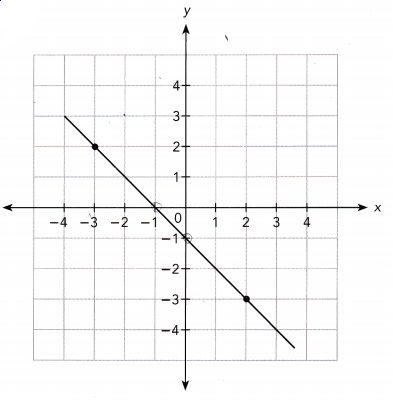The line passes through the points (0, -1) and (2, -3).
Slope m = $$\frac{-3-(-1)}{2-0}$$
= $$\frac{-2}{2}$$
= -1
The line intersects the y-axis at the point (0, -1).
So, the y-intercept is -1.
So, the equation of the line is y = -1x -1.

Question 3.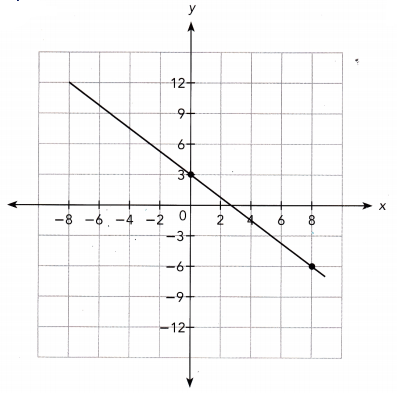The line passes through the points (0, 3) and (8, -6).
Slope m = $$\frac{-6-3}{8-0}$$
= $$\frac{-9}{8}$$
m = $$\frac{-9}{8}$$
The line intersects the y-axis at the point (0, 3).
So, the y-intercept is 3.

Question 4.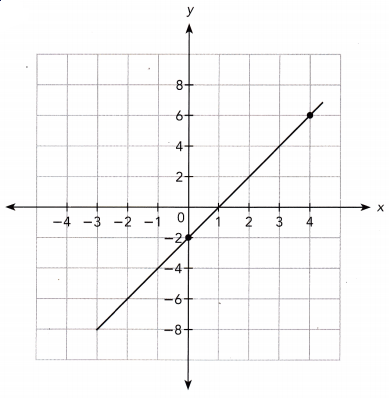The line passes through the points (0, 2) and (4, 6).
Slope m = $$\frac{6-2}{4-0}$$
= $$\frac{4}{4}$$
m = 1
The line intersects the y-axis at the point (0, -2).
So, the y-intercept is -2.

Write an equation in the form y = mx or y = mx + b for each line.

Question 5.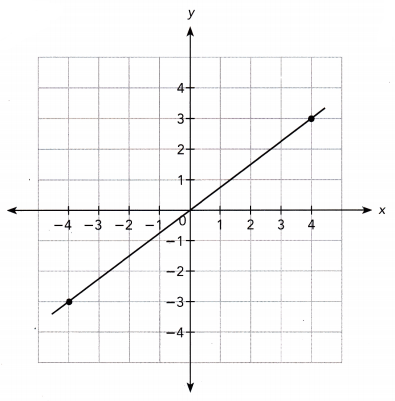The line passes through the points (4, 3) and (-4, -3).
Slope m = $$\frac{-3-3}{-4-4}$$
= $$\frac{-6}{-8}$$
= $$\frac{3}{4}$$
The line intersects the y-axis at the point (0, 0).
So, the y-intercept is 0.
So, the equation of the line is y = $$\frac{3}{4}$$x

Question 6.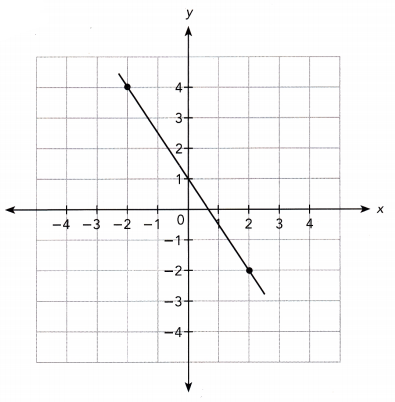The line passes through the points (-2, 4) and (2, -2).
Slope m = $$\frac{-2-4}{2+2}$$
= $$\frac{-6}{4}$$
= $$\frac{-3}{2}$$
The line intersects the y-axis at the point (0, 1).
So, the y-intercept is 1.
So, the equation of the line is y = $$\frac{-3}{2}$$x +1.

Question 7.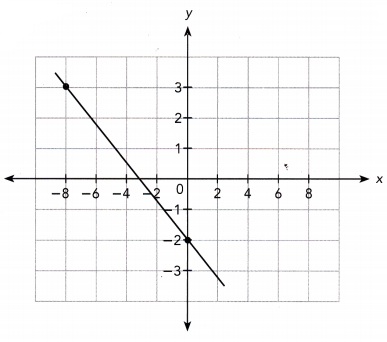The line passes through the points (-8, 3) and (0, -2).
Slope m = $$\frac{-2-3}{0+8}$$
= $$\frac{-5}{8}$$
The line intersects the y-axis at the point (0, -2).
So, the y-intercept is -2.
So, the equation of the line is y = $$\frac{-5}{8}$$x -2.

Question 8.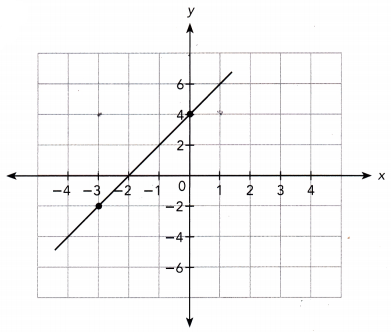The line passes through the points (0, 4) and (-3, -2).
Slope m = $$\frac{-2-4}{-3-0}$$
= $$\frac{-6}{-3}$$
= 2
The line intersects the y-axis at the point (0, 4).
So, the y-intercept is 4.
So, the equation of the line is y = 2x + 4

Graph each line using 1 grid square to represent 1 unit on both axes for the interval from -4 to 4. Then write the equation for each line.

Question 9.
The line passes through the points (-4, 3) and (-4, -2).